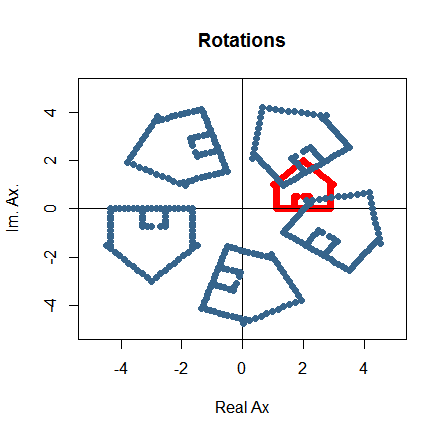### PLOTTING IN THE COMPLEX PLANE:

The code in R can be found here.

The $$x$$ coordinate is the real part, and the $$y$$ coordinate, the imaginary part.

Translation can be achieved by adding a complex number to every point. Scaling is done by multiplying by a scalar.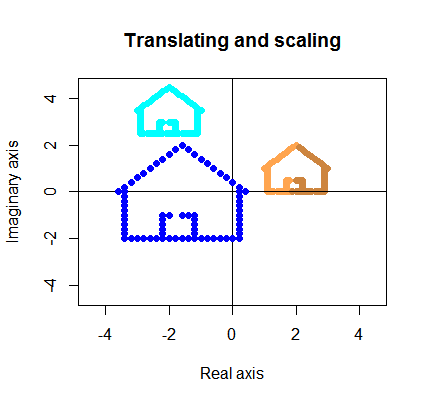Horizontal and vertical flips require separating the real from the imaginary components before multplying either the real part by $$-1$$ to achieve a horizontal flip; or the imaginary part by $$-1$$ for a vertical flip: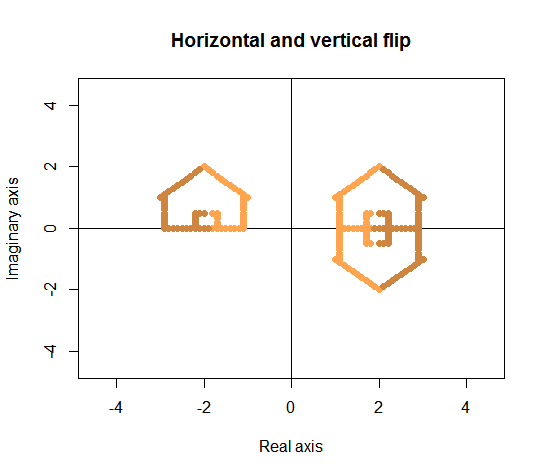Inverting is accomplished simply multiplying by the scalar $$-1$$, and the way to picture it is by realizding that the $$-1$$ changes the the sign of the real and imaginary components.

To rotate $$90$$ degrees we multiply by $$i$$:

$$i \times (x + y*i) = -y +x*i.$$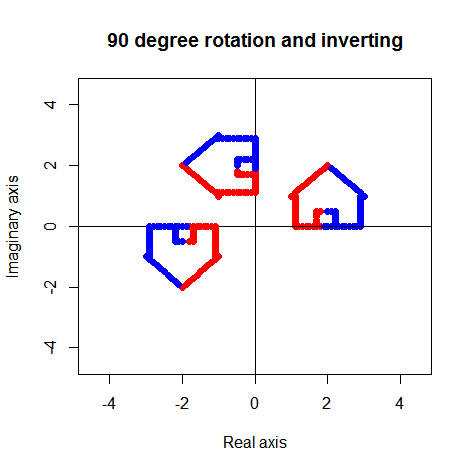Rotating by another angle requires some background:

Argument of a complex angle: angle between the vectorial representation of the complex number and $$1 + 0i.$$ This correponds to the angle, measured in radians, between the x axis and the arrow representing the complex number.

Euler’s formula codes every point in the unit circle as:

$$\Large e^{\theta\,i}$$ with $$\large \theta$$ being the argument.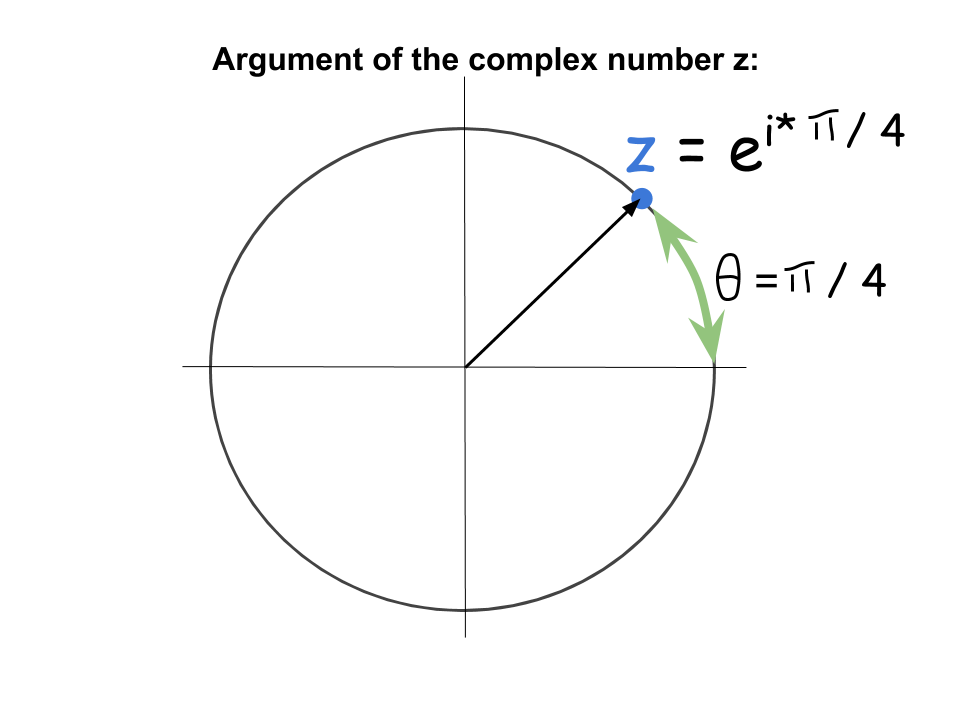The trick is to divide the circle $$2\,\pi$$ into an arbritary number of equally spaced points, for instance, $$200$$: $$2\,\pi/200$$. In this way, every point can be rotated a given amount of these divisions of the circle (e.g. $$n$$) as follows (Euler’s formula):

$$\Huge e^{\,\huge i\times\,n \,\times \frac{2\pi}{200}}= \Large cos(n \,\times \frac{2\pi}{200}) + i\,sin(n \,\times \frac{2\pi}{200}).$$

The code in R is:

exp(#_of_"slices" * 1i * (2*pi / total_number_slices)) * complex_#s